The OpenFOAM Foundation
atmBoundaryLayer Class Reference

This class provides functions to evaluate the velocity and turbulence distributions appropriate for atmospheric boundary layers (ABL). More...

Inheritance diagram for atmBoundaryLayer: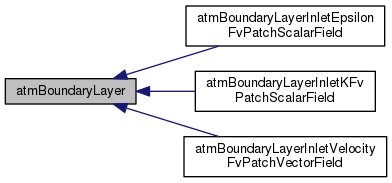[legend]

## Public Member Functions

atmBoundaryLayer ()
Construct null. More...

atmBoundaryLayer (const vectorField &p, const dictionary &)
Construct from the coordinates field and dictionary. More...

atmBoundaryLayer (const atmBoundaryLayer &, const fvPatchFieldMapper &)
Construct by mapping given. More...

atmBoundaryLayer (const atmBoundaryLayer &)
Construct as copy. More...

const vectorflowDir () const
Return flow direction. More...

const vectorzDir () const
Return z-direction. More...

const scalarFieldUstar () const
Return friction velocity. More...

void autoMap (const fvPatchFieldMapper &)
Map (and resize as needed) from self given a mapping object. More...

void rmap (const atmBoundaryLayer &, const labelList &)
Reverse map the given fvPatchField onto this fvPatchField. More...

tmp< vectorFieldU (const vectorField &p) const
Return the velocity distribution for the ATM. More...

tmp< scalarFieldk (const vectorField &p) const
Return the turbulent kinetic energy distribution for the ATM. More...

tmp< scalarFieldepsilon (const vectorField &p) const
Return the turbulent dissipation rate distribution for the ATM. More...

void write (Ostream &) const
Write. More...

## Detailed Description

This class provides functions to evaluate the velocity and turbulence distributions appropriate for atmospheric boundary layers (ABL).

The profile is derived from the friction velocity, flow direction and "vertical" direction: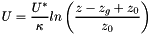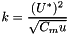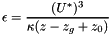where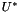= Friction velocity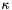= von Karman's constant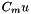= Turbulence viscosity coefficient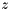= Vertical coordinate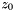= Surface roughness height [m]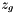= Minimum z-coordinate [m]

and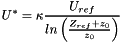where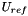= Reference velocity at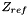[m/s]= Reference height [m]

Use in the atmBoundaryLayerInletVelocity, atmBoundaryLayerInletK and atmBoundaryLayerInletEpsilon boundary conditions.

Reference: D.M. Hargreaves and N.G. Wright, "On the use of the k-epsilon model in commercial CFD software to model the neutral atmospheric boundary layer", Journal of Wind Engineering and Industrial Aerodynamics 95(2007), pp 355-369.

Usage
Property Description Required Default
flowDir Flow direction yes
zDir Vertical direction yes
kappa von Karman's constant no 0.41
Cmu Turbulence viscosity coefficient no 0.09
Uref Reference velocity [m/s] yes
Zref Reference height [m] yes
z0 Surface roughness height [m] yes
zGround Minimum z-coordinate [m] yes

Example of the boundary condition specification:

    ground
{
type            atmBoundaryLayerInletVelocity;
flowDir         (1 0 0);
zDir            (0 0 1);
Uref            10.0;
Zref            20.0;
z0              uniform 0.1;
zGround         uniform 0.0;
}
Note
D.M. Hargreaves and N.G. Wright recommend Gamma epsilon in the k-epsilon model should be changed from 1.3 to 1.11 for consistency. The roughness height (Er) is given by Er = 20 z0 following the same reference.
Source files

Definition at line 205 of file atmBoundaryLayer.H.

## Constructor & Destructor Documentation

 atmBoundaryLayer ( )

Construct null.

Definition at line 35 of file atmBoundaryLayer.C.

Referenced by atmBoundaryLayer::atmBoundaryLayer().

Here is the caller graph for this function: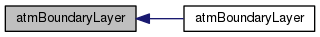atmBoundaryLayer ( const vectorField & p, const dictionary & dict )

Construct from the coordinates field and dictionary.

Definition at line 49 of file atmBoundaryLayer.C.

Here is the call graph for this function: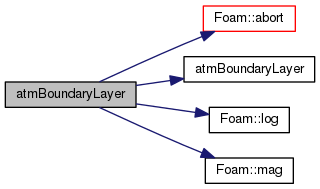atmBoundaryLayer ( const atmBoundaryLayer & ptf, const fvPatchFieldMapper & mapper )

Construct by mapping given.

atmBoundaryLayer onto a new patch

Definition at line 77 of file atmBoundaryLayer.C.

 atmBoundaryLayer ( const atmBoundaryLayer & blpvf )

Construct as copy.

Definition at line 94 of file atmBoundaryLayer.C.

## Member Function Documentation

 const vector& flowDir ( ) const
inline

Return flow direction.

Definition at line 264 of file atmBoundaryLayer.H.

 const vector& zDir ( ) const
inline

Return z-direction.

Definition at line 270 of file atmBoundaryLayer.H.

 const scalarField& Ustar ( ) const
inline

Return friction velocity.

Definition at line 276 of file atmBoundaryLayer.H.

Here is the call graph for this function: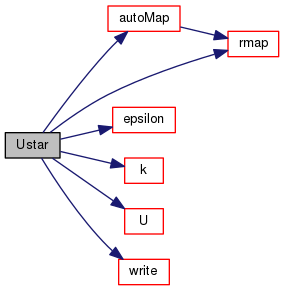void autoMap ( const fvPatchFieldMapper & m )

Map (and resize as needed) from self given a mapping object.

Definition at line 110 of file atmBoundaryLayer.C.

References Field< Type >::autoMap(), and atmBoundaryLayer::rmap().

Here is the call graph for this function: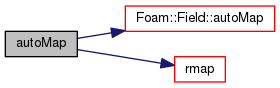Here is the caller graph for this function: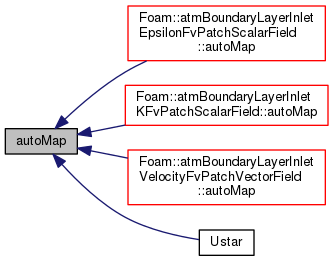void rmap ( const atmBoundaryLayer & blptf, const labelList & addr )

Reverse map the given fvPatchField onto this fvPatchField.

Definition at line 119 of file atmBoundaryLayer.C.

References Field< Type >::rmap().

Here is the call graph for this function: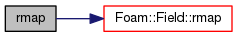Here is the caller graph for this function: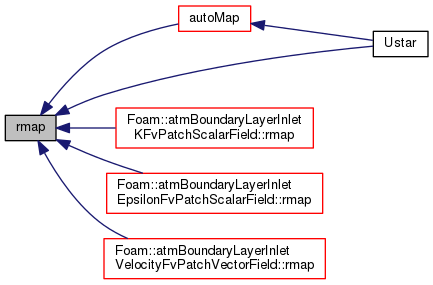tmp< vectorField > U ( const vectorField & p ) const

Return the velocity distribution for the ATM.

Definition at line 130 of file atmBoundaryLayer.C.

References Foam::log().

Referenced by atmBoundaryLayer::Ustar().

Here is the call graph for this function: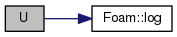Here is the caller graph for this function: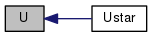tmp< scalarField > k ( const vectorField & p ) const

Return the turbulent kinetic energy distribution for the ATM.

Definition at line 142 of file atmBoundaryLayer.C.

References Foam::sqr(), and Foam::sqrt().

Referenced by atmBoundaryLayer::Ustar().

Here is the call graph for this function: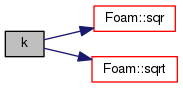Here is the caller graph for this function: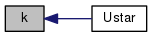tmp< scalarField > epsilon ( const vectorField & p ) const

Return the turbulent dissipation rate distribution for the ATM.

Definition at line 148 of file atmBoundaryLayer.C.

References p, and Foam::pow3().

Referenced by atmBoundaryLayer::Ustar().

Here is the call graph for this function: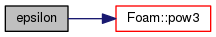Here is the caller graph for this function: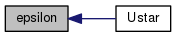void write ( Ostream & os ) const

Write.

Definition at line 154 of file atmBoundaryLayer.C.

Here is the call graph for this function: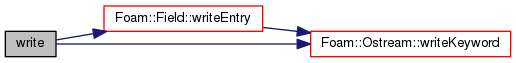Here is the caller graph for this function: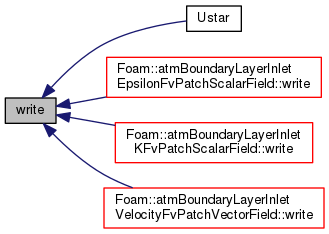The documentation for this class was generated from the following files:
• src/TurbulenceModels/turbulenceModels/RAS/derivedFvPatchFields/atmBoundaryLayer/atmBoundaryLayer.H
• src/TurbulenceModels/turbulenceModels/RAS/derivedFvPatchFields/atmBoundaryLayer/atmBoundaryLayer.C